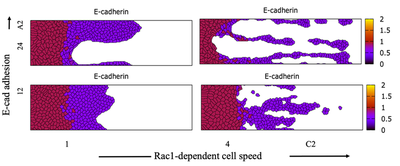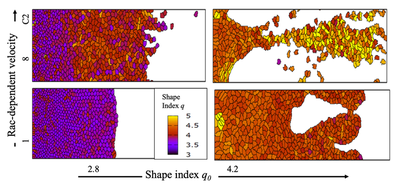# MDCK EMT C

##### Persistent Identifier

Multiple models in epithelial-mesenchymal transitions (EMT)

## Introduction

We model epithelial-mesenchymal transition (EMT) by first assembling an ODE model for intracellular Yes-associated protein (YAP) signalling and then embedding this single cell model within individual cells in a multiscale simulation. This page contains XML files of the models investigated in the paper by Mukhtar et al. (2022).

## Description

### Effect of Adhesion and Cell Speed

Park et al. (2019) showed experimentally that blocking the adhesive function of E-cadherin with antibodies led to less stable fingers and more breakage of cells and clusters than in control experiments. The following figure shows that simultaneously varying E-cadherin-dependent adhesion (A2) and Rac-1-dependent cell speed (C2) has significant effect on sheet morphology. Cell sheets expand even at low C2, driven by pressure from cell division at the back. Increasing cell speed and lowering adhesion correlate with longer, more fragile fingers, and massive dissemination. Stronger adhesion favors more stable, long sheet protrusions. Interestingly, low cell speed (C2 = 1, left column) can be compensated by high adhesion (A2 = 24, top left) in enabling invasive growth. As this sequence indicates, a strong adhesive force between cells can help to combine weak individual cell-migratory forces into a single collective group force that pushes a large sheet protrusion outward. The two extremes illustrate the dichotomy between rapid metastasis versus stable collective invasion.Effect of cell-cell E-cadherin-dependent adhesion (A2) and Rac1-dependent cell speed (C2) on sheet morphology. All images at $t = 1500\ \text{MCS}$. Produced with C2vsA2_main.xml (with cell division restricted to cell volume). Color depicts the relative level of E-cadherin in each cell.

### Effect of Cell Biophysical Properties

Biophysical properties of the cells effect cell morphology. The ‘cell shape index’ $q_0 = p_0/\sqrt{a_0}$ was adopted to represent the cell’s preferred shape in 2D, and $q = p/\sqrt{a}$ represents the cell’s actual shape index, where $p_0$ is the cell’s preferred perimeter, $a_0$ is the cell’s preferred area, $p$ is the cell’s actual perimeter and $a$ is the cell’s actual area. As shown in the following figure, low speed and preferred shape index corresponds to sluggish, solid-like ‘jammed’ behaviour and a relatively flat leading edge. Increasing $q_0$ at low speed results in more fluid, fat fingering. Keeping $q_0$ low while increasing cell speed accelerates expansion, with minimal fingering. Increasing both $q_0$ and the cell speed leads to thinner, faster fingers, with eventual breakage and massive dissemination.Effect of the preferred cell shape index $q_0$ and the Rac1-dependent cell speed C2 on sheet morphology. As in the main text Fig. 6, but with cell division allowed only for cells with cell.volume > 40. At lower left, most cells are ‘jammed’ (purple). At upper right, the sheet is fluid, with finer, long, fast-growing fingers. Produced with BiPlot.xml. All results are at $t = 1500\ \text{MCS}$.

## Results

Video of the model simulation C2vsA2_main.xml: Effect of cell-cell E-cadherin-dependent adhesion (here A2 = 12) and Rac1-dependent cell speed (here C2 = 4) on sheet morphology (with cell division restricted to cell.volume > 40). Color depicts the relative level of E-cadherin in each cell (see Fig. 5 in Mukhtar et al. (2022)).

Video of the model simulation qvsC2.xml: Default simulation on the effect of the preferred shape index, $q_0 = \frac{p_0}{\sqrt{a_0}}$ and the Rac1-dependent cell speed, C2, on sheet morphology. The color map depicts the individual cells’ shape index $q$. The default simulation has C2 = 4 and $q_0$ resulting from the aspherity attributes strength = 1 and target = 1 (see Fig. 6 in Mukjtar et al. (2022)).

## Reference

This model is the original used in the publication, up to technical updates:

N. Mukhtar, E. N. Cytrynbaum, L. Edelstein-Keshet: A multiscale computational model of YAP signaling in epithelial fingering behavior. Biophys. J. 121 (10): 1940-1948, 2022.

## Model

Get this model via:

•  Download: C2vsA2_main.xml
• XML Preview

<?xml version='1.0' encoding='UTF-8'?>
<MorpheusModel version="4">
<Description>
<Details>Naba Mukhtar, Eric N Cytrynbaum, and Leah Edelstein-Keshet (2022) A Multiscale computational model of YAP signaling in epithelial fingering behaviour

This xml file was used to produce Figure 5, by varying A2 = 6,12,24
and C2 = 1,4,8.

Similar to the file YREsheetNRA.xml, but with cell division restricted to cells beyond some size.

We thank Lutz Brusch for providing a basic cell sheet simulation that we modified and adapted to this project </Details>
<Title>YREsheetNRAShapeFactorq</Title>
</Description>
<Space>
<Lattice class="square">
<Neighborhood>
<Order>2</Order>
</Neighborhood>
<Size symbol="size" value="400, 100, 0"/>
<BoundaryConditions>
<Condition boundary="x" type="constant"/>
<Condition boundary="-x" type="noflux"/>
<Condition boundary="y" type="periodic"/>
<Condition boundary="-y" type="periodic"/>
</BoundaryConditions>
</Lattice>
<SpaceSymbol symbol="space"/>
</Space>
<Time>
<StartTime value="0"/>
<StopTime symbol="stoptime" value="1500"/>
<TimeSymbol symbol="time"/>
<RandomSeed value="1"/>
</Time>
<Analysis>
<Gnuplotter time-step="50" decorate="true">
<Terminal name="png"/>
<!--    <Disabled>
<Plot>
<Cells max="5" value="q" min="3" flooding="true">
<Disabled>
<ColorMap>
<Color color="lemonchiffon" value="0"/>
<Color color="light-blue" value="0.05"/>
<Color color="light-red" value="0.1"/>
</ColorMap>
</Disabled>
</Cells>
</Plot>
</Disabled>
-->
<!--    <Disabled>
<Plot title="Rac1">
<Cells max="3" value="R" min="0" flooding="true">
<ColorMap>
<Color color="blue" value="0"/>
<Color color="red" value="3"/>
<Color color="yellow" value="4"/>
</ColorMap>
</Cells>
</Plot>
</Disabled>
-->
<Plot>
<Cells max="2" value="E" min="0" flooding="true">
<Disabled>
<ColorMap>
<Color color="plum" value="0"/>
<Color color="blue" value="4"/>
<Color color="cyan" value="7"/>
</ColorMap>
</Disabled>
</Cells>
</Plot>
<!--    <Disabled>
<Plot>
<Cells value="d.abs" flooding="true">
<Disabled>
<ColorMap>
<Color color="skyblue" value="0"/>
<Color color="violet" value="0.1"/>
<Color color="salmon" value="0.2"/>
</ColorMap>
</Disabled>
</Cells>
</Plot>
</Disabled>
-->
<!--    <Disabled>
<Plot>
<Cells value="Cr" flooding="true">
<Disabled>
<ColorMap>
<Color color="red" value="0"/>
<Color color="red" value="0"/>
</ColorMap>
</Disabled>
</Cells>
</Plot>
</Disabled>
-->
</Gnuplotter>
<!--    <Disabled>
<Logger time-step="1.0">
<Input>
<Symbol symbol-ref="cell.center.x"/>
<Symbol symbol-ref="cell.center.y"/>
<Symbol symbol-ref="q"/>
</Input>
<Output>
<TextOutput/>
</Output>
<Plots>
<Plot time-step="-1">
<Style style="points" point-size="0.05"/>
<Terminal terminal="png"/>
<X-axis maximum="size.x" minimum="0.0">
<Symbol symbol-ref="cell.center.x"/>
</X-axis>
<Y-axis maximum="stoptime" minimum="0">
<Symbol symbol-ref="time"/>
</Y-axis>
<Color-bar maximum="5" palette="default" minimum="3">
<Symbol symbol-ref="q"/>
</Color-bar>
</Plot>
</Plots>
</Logger>
</Disabled>
-->
<ModelGraph include-tags="#untagged" reduced="false" format="dot"/>
</Analysis>
<Global>
<Constant symbol="ky" value="0.1" name="Basal rate of YAP activation"/>
<Constant symbol="kye" value="1.8" name="E-cadherin-dependent rate of YAP deactivation"/>
<Constant symbol="Dy" value="2" name="Inactivation rate of YAP"/>
<Constant symbol="C" value="0.9" name="Initial activation rate of E-cadherin"/>
<Constant symbol="ke" value="0.9" name="YAP-dependent rate of E-cadherin expression"/>
<Constant symbol="K" value="1" name="Dissociation constant of YAP-WT1 transcriptional constant"/>
<Constant symbol="De" value="1" name="Inactivation rate of E-cadherin"/>
<Constant symbol="h" value="3" name="Hill coefficient for E-cadherin"/>
<Constant symbol="kr" value="1" name="YAP-dependent rate of Rac1 expression"/>
<Constant symbol="Kr" value="0.5" name="Michaelis-Menten-like constant for Rac1"/>
<Constant symbol="Dr" value="0.5" name="Degradation rate of Rac1"/>
<Constant symbol="n" value="6" name="Hill coefficient for Rac1"/>
<Constant symbol="alphaR" value="1" name="Rac activation fraction"/>
<Constant symbol="kyr" value="1.8" name="Rac1-dependent rate of YAP activation"/>
<!--    <Disabled>
</Constant>
</Disabled>
-->
<Annotation>Control</Annotation>
</Constant>
<Constant symbol="A3" value="0.85" name="E-cadherin half &quot;saturation&quot; "/>
<Constant symbol="C1" value="0.4" name="basal migration"/>
<Constant symbol="C2" value="4" name="Max Rac1 migration constant"/>
<Constant symbol="C3" value="3" name="Rac1 half &quot;saturation&quot;"/>
<Constant symbol="tlim" value="100" name="max time for initial leader cells to emerge"/>
<Constant symbol="frac" value="0.2" name="fraction of neighbourhood Cr that the cell receives each time Cr spreads to it"/>
<!--    <Disabled>
<Constant symbol="Ytot" value="0.5" name="Total YAP (active (Y) + inactive)">
<Annotation>KD</Annotation>
</Constant>
</Disabled>
-->
<Constant symbol="Ytot" value="1.2" name="Total YAP (active (Y) + inactive)">
<Annotation>Control</Annotation>
</Constant>
<!--    <Disabled>
<Constant symbol="Ytot" value="2" name="Total YAP (active (Y) + inactive)">
<Annotation>OE</Annotation>
</Constant>
</Disabled>
-->
<Constant symbol="Rtot" value="5" name="Total Rac1 (active (R) + inactive)"/>
<Constant symbol="shareprob" value="0.02" name="parameter that determines probability of Cr spread in each time step"/>
<Variable symbol="Cr" value="0.0" name="Basal Rac1 activation rate"/>
<!--    <Disabled>
<Variable symbol="x_edge2" value="0.0"/>
</Disabled>
-->
<!--    <Disabled>
<Mapper time-step="1.0" name="leftmost edge cell">
<Input value="(M>0)*cell.center.x*(cell.center.x>10) + (M==0)*size.x + (M>0)*size.x*(cell.center.x&lt;=10)"/>
<Output mapping="minimum" symbol-ref="x_edge2"/>
</Mapper>
</Disabled>
-->
</Global>
<CellTypes>
<CellType name="medium" class="medium"/>
<CellType name="dividingcell" class="biological">
<VolumeConstraint strength="1" target="50"/>
<ConnectivityConstraint/>
<SurfaceConstraint strength="1" target="1" mode="aspherity"/>
<CellDivision division-plane="major">
<Condition>(rand_uni(0,1)&lt;0.006*(exp(-time/300)+0.2)) * (cell.center.x&lt;40) * (cell.volume>40)</Condition>
<Triggers/>
<Annotation>Only cells in the first 40 pixels can divide, regardless of domain size. Before, this was size.x*0.1</Annotation>
</CellDivision>
<CellDeath name="delete cells near right domain edge">
<Condition>(cell.center.x>0.99*size.x)</Condition>
</CellDeath>
<DirectedMotion strength="C1+C2*R/(C3+R)" direction="1, 0.0, 0.0" name="cell migration"/>
<Property symbol="Y" value="0" name="YAP"/>
<Property symbol="R" value="0" name="Rac1"/>
<Property symbol="Cr" value="0.001"/>
<Property symbol="dist" value="0.0" name="distance from right domain edge"/>
<Property symbol="avspeed" value="0.0" name="Sum of instantaneous speeds each time step (times 100)"/>
<Property symbol="truavspeed" value="0.0" name="Average Speed * 100"/>
<Property symbol="avspeed2" value="0.0" name="Sum of instantaneous speeds over final 200 time steps (times 100)"/>
<Property symbol="truavspeed2" value="0.0" name="average speed over final 200 time steps (times 100)"/>
<Property symbol="M" value="0.0" name="Contact with medium"/>
<Property symbol="neigh" value="0.0" name="number of neighbour cells"/>
<Property symbol="av" value="0.0" name="average neighbourhood Cr"/>
<PropertyVector symbol="d" value="0.0, 0.0, 0.0" name="speed"/>
<NeighborhoodReporter>
<Input value="cell.type == celltype.medium.id" scaling="length"/>
<Output mapping="sum" symbol-ref="M"/>
</NeighborhoodReporter>
<NeighborhoodReporter>
<Input value="cell.type == celltype.dividingcell.id" scaling="cell"/>
<Output mapping="sum" symbol-ref="neigh"/>
</NeighborhoodReporter>
<NeighborhoodReporter>
<Input value="Cr" scaling="cell"/>
<Output mapping="average" symbol-ref="av"/>
</NeighborhoodReporter>
<MotilityReporter time-step="50">
<Velocity symbol-ref="d"/>
</MotilityReporter>
<DiffEqn name="Equation for YAP" symbol-ref="Y">
<Expression>(ky+kyr*R)*(Ytot-Y) - (kye*Y*E + Dy*Y)</Expression>
</DiffEqn>
<Expression>C - ke*Y^h/(K^h+Y^h) - De*E</Expression>
</DiffEqn>
<DiffEqn name="Equation for Rac1" symbol-ref="R">
<Expression>alphaR*(Cr + kr*Y^n/(Kr^n+Y^n))*(Rtot-R) - Dr*R</Expression>
</DiffEqn>
<Rule name="Equation for Cr spread; at each time step before tlim, cells in contact with the medium have a small chance of being endowed with high Cr; at each time step, each cell has a small chance of having its Cr be augmented by the average neighbourhood Cr (up to a max value); cells at the left edge of the domain retain low Cr" symbol-ref="Cr">
<Expression>Cr+(0.1*(M>8)*(rand_uni(0,1)&lt;0.008)*(time&lt;tlim)+frac*av*(rand_uni(0,1)&lt;shareprob))*(Cr&lt;0.1)*(cell.center.x>20)</Expression>
</Rule>
<Rule name="distance from right domain edge" symbol-ref="dist">
<Expression>size.x - cell.center.x</Expression>
</Rule>
<Rule name="Sum of instantaneous speeds each time step (times 100)" symbol-ref="avspeed">
<Expression>avspeed+d.abs*100</Expression>
</Rule>
<Rule name="average speed over the simulation (times 100)" symbol-ref="truavspeed">
<Expression>avspeed/time</Expression>
</Rule>
<Rule name="Sum of instantaneous speeds over final 100 time steps (times 100)" symbol-ref="avspeed2">
<Expression>avspeed2 + d.abs*100*(time > stoptime-201)</Expression>
</Rule>
<Rule name="average speed over 100 time steps (times 100)" symbol-ref="truavspeed2">
<Expression>avspeed2/200</Expression>
</Rule>
<Rule symbol-ref="q">
<Expression>cell.surface/sqrt(cell.volume)</Expression>
</Rule>
<!--    <Disabled>
<Rule symbol-ref="q">
<Expression>cell.volume</Expression>
</Rule>
</Disabled>
-->
</System>
<Property symbol="q" value="0.0" name="shape index"/>
</CellType>
</CellTypes>
<CellPopulations>
<Population name="Initialize cell sheet at left edge of the domain" type="dividingcell" size="1">
<!--    <Disabled>
<InitRectangle number-of-cells="70" mode="regular">
<Dimensions origin="size.x/100, 0, 0" size="size.x/100, size.y, size.z"/>
</InitRectangle>
</Disabled>
-->
<InitRectangle number-of-cells="70" mode="regular">
<Dimensions origin="0.0, 0.0, 0.0" size="4.0, size.y, 0.0"/>
</InitRectangle>
</Population>
</CellPopulations>
<CPM>
<Interaction>
<Contact type2="dividingcell" type1="dividingcell" value="30">
</Contact>
<Contact type2="medium" type1="dividingcell" value="12"/>
</Interaction>
<ShapeSurface scaling="norm">
<Neighborhood>
<Order>2</Order>
</Neighborhood>
</ShapeSurface>
<MonteCarloSampler stepper="edgelist">
<MCSDuration value="1"/>
<MetropolisKinetics temperature="1"/>
<Neighborhood>
<Order>2</Order>
</Neighborhood>
</MonteCarloSampler>
</CPM>
</MorpheusModel>



• C2vsA2_main.xml (produced Fig. 5),
• qvsC2.xml (produced Fig. 6) and
• BiPlot.xml (produced SI Fig. S11),

see the referenced paper as well as supplemental information.

For information on models

• Biophys.xml (produced SI Fig. S12 by varying parameters SurfaceConstraint strength and the VolumeConstraint strength),
• NRAEcadCntr.xml (produced SI Fig. S8 by varying parameter A2) and
• NRARac1Cntr.xml (produced SI Fig. S8 by varying parameter $\alpha$)

which are here attached but not discussed, please refer to the supplemental information of the referenced paper.